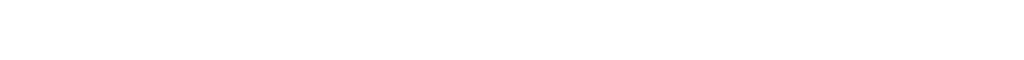17.1.1 Applying the Equilibrium Law

Explaining Le Châtelier’s Principle

Le Châtelier’s principle

• Le Châtelier’s principle says that if a change is made to a system at dynamic equilibrium, the position of the equilibrium moves to minimise this change
• The principle can be used to predict changes to the position of equilibrium when there are changes in temperature, pressure or concentration

Explaining Le Châtelier’s Principle

• The equilibrium law can explain and quantify the effect of changes in concentration at a particular temperature
• These explanations are based on the idea that Kc is not affected by a change in concentration
• Remember that the position of equilibrium is affected by a change in concentration:

Effects of Concentration Table• If the concentration of A increases
• The position of equilibrium shifts to the right as the forward reaction works to remove A
• The concentrations of C and D increase to minimise this change
• The concentration of B decreases because it is being used up to minimise the change
• Therefore, the value of Kc remains unchanged
• If the concentration of A decreases
• The position of equilibrium shifts to the left as the backward reaction works to replace A
• The concentrations of C and D decrease to minimise this change
• The concentration of B increases because it is also being produced when C and D react
• Therefore, the value of Kc remains unchanged
• The Haber Process is represented by the following chemical equation:

N2 (g) + 3H2 (g) ⇌ 2NH3 (g)

• An increase in the amount of nitrogen will cause the following:
• The equilibrium to shift to the right
• An increase in the amount of ammonia
• A decrease in the amount of hydrogen
• Kc will remain unchanged

Graph showing the effects of adding nitrogen on the concentration of reactants and products in the Haber Process

• An increase in the amount of nitrogen causes the rate of the forward reaction to increase
• This means that more ammonia is produced, causing the rate of the backward reaction to increase
• This process of increasing forward and backward reactions continues until a new equilibrium is established
• The rate at this newly established equilibrium will be higher than the original rate

Graph showing the effects of adding nitrogen on the rate of reaction in the Haber Process

• Similar points about concentrations and rates can be made for the addition of hydrogen or the removal of ammonia
• Regardless, the value of Kc remains unchanged
• Only changes in temperature affect Kc

Equilibrium Problems

Calculations involving Kc

• In the equilibrium expression, each term inside a square bracket represents the concentration of that chemical in mol dm-3
• Therefore, the units of Kc depend on the equilibrium expression
• Some questions give the number of moles of each of the reactants and products at equilibrium together with the volume of the reaction mixture
• The concentrations of the reactants and products can then be calculated from the number of moles and total volumeEquation to calculate concentration from number of moles and volume

Worked Example

Calculating Kc of ethanoic acid

Ethanoic acid and ethanol react to form the ester ethyl ethanoate and water as follows:

CH3COOH (I) + C2H5OH (I) ⇌ CH3COOC2H5 (I) + H2O (I)

At equilibrium, 500 cm3 of the reaction mixture contained 0.235 mol of ethanoic acid and 0.035 mol of ethanol together with 0.182 mol of ethyl ethanoate and 0.182 mol of water

Use this data to calculate a value of Kc for this reaction

Step 1: Calculate the concentrations of the reactants and products:Step 2: Write out the balanced symbol equation with the concentrations of each chemical underneath:Step 3: Write out the equilibrium constant for the reaction:Step 4: Substitute the equilibrium concentrations into the expression and calculate the answer:Step 5: Deduce the correct units for Kc:• All units cancel out
• Therefore, Kc = 4.03
• Note that the smallest number of significant figures used in the question is 3, so the final answer should also be given to 3 significant figures
• Some questions give the initial and equilibrium concentrations of the reactants but not the products
• An initial, change and equilibrium (ICE) table should be used to determine the equilibrium concentration of the products using the molar ratio of reactants and products in the stoichiometric equation

Worked Example

Calculating Kc of ethyl ethanoate

Ethyl ethanoate is hydrolysed by water:

CH3COOC2H5 (I) + H2O (I) ⇌ CH3COOH (I) + C2H5OH (I)

0.1000 mol of ethyl ethanoate are added to 0.1000 mol of water. A little acid catalyst is added and the mixture made up to 1 dm3. At equilibrium 0.0654 mol of water are present.

Use this data to calculate a value of Kc for this reaction.

Step 1: Write out the balanced chemical equation with the concentrations of beneath each substance using an initial, change and equilibrium table:Step 2: Calculate the concentrations of the reactants and products:CloseClose